#### Convergence issues

If the maximum number of stages is fixed in the problem definition, then convergence is assured. Suppose, however, that there is no limit on the number of stages. Recall from Section 2.3.2 that each value iteration increases the total path length by one. The actual stage indices were not important in backward dynamic programming because arbitrary shifting of indices does not affect the values. Eventually, the algorithm terminated because optimal cost-to-go values had been computed for all reachable states from the goal. This resulted in a stationary cost-to-go function because the values no longer changed. States that are reachable from the goal converged to finite values, and the rest remained at infinity. The only problem that prevents the existence of a stationary cost-to-go function, as mentioned in Section 2.3.2, is negative cycles in the graph. In this case, the best plan would be to loop around the cycle forever, which would reduce the cost to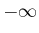.

In the current setting, a stationary cost-to-go function once again arises, but cycles once again cause difficulty in convergence. The situation is, however, more complicated due to the influence of nature. It is helpful to consider a plan-based state transition graph,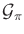. First consider the nondeterministic case. If there exists a plan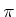from some state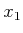for which all possible actions of nature cause the traversal of cycles that accumulate negative cost, then the optimal cost-to-go atconverges to, which prevents the value iterations from terminating. These cases can be detected in advance, and each such initial state can be avoided (some may even be in a different connected component of the state space).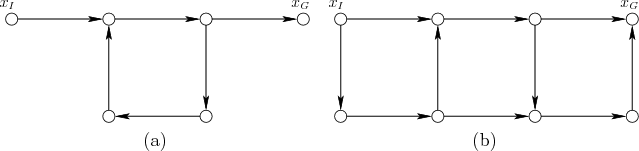It is also possible that there are unavoidable positive cycles. In Section 2.3.2, the cost-to-go function behaved differently depending on whether the goal set was reachable. Due to nature, the goal set may be possibly reachable or guaranteed reachable, as illustrated in Figure 10.2. To be possibly reachable from some initial state, there must exist a plan,, for which there exists a sequence of nature actions that will lead the state into the goal set. To be guaranteed reachable, the goal must be reached in spite of all possible sequences of nature actions. If the goal is possibly reachable, but not guaranteed reachable, from some stateand all edges have positive cost, then the cost-to-go value oftends to infinity as the value iterations are repeated. For example, every plan-based state transition graph may contain a cycle of positive cost, and in the worst case, nature may cause the state to cycle indefinitely. If convergence of the value iterations is only evaluated at states from which the goal set is guaranteed to be reachable, and if there are no negative cycles, then the algorithm should terminate when all cost-to-go values remain unchanged.

For the probabilistic case, there are three situations:

1. The value iterations arrive at a stationary cost-to-go function after a finite number of iterations.
2. The value iterations do not converge in any sense.
3. The value iterations converge only asymptotically to a stationary cost-to-go function. The number of iterations tends to infinity as the values converge.
The first two situations have already occurred. The first one occurs if there exists a plan,, for whichhas no cycles. The second situation occurs if there are negative or positive cycles for which all edges in the cycle have probability one. This situation is essentially equivalent to that for the nondeterministic case. Worst-case analysis assumes that the worst possible nature actions will be applied. For the probabilistic case, the nature actions are forced by setting their probabilities to one.

The third situation is unique to the probabilistic setting. This is caused by positive or negative cycles infor which the edges have probabilities in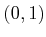. The optimal plan may even have such cycles. As the value iterations consider longer and longer paths, a cycle may be traversed more times. However, each time the cycle is traversed, the probability diminishes. The probabilities diminish exponentially in terms of the number of stages, whereas the costs only accumulate linearly. The changes in the cost-to-go function gradually decrease and converge only to stationary values as the number of iterations tends to infinity. If some approximation error is acceptable, then the iterations can be terminated once the maximum change over all ofis within some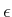threshold. The required number of value iterations to obtain a solution of the desired quality depends on the probabilities of following the cycles and on their costs. If the probabilities are lower, then the algorithm converges sooner.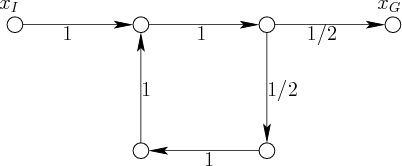Example 10..6 (A Cycle in the Transition Graph)   Suppose that a plan,, is chosen that yields the plan-based state transition graph shown in Figure 10.3. A probabilistic model is used, and the probabilities are shown on each edge. For simplicity, assume that each transition results in unit cost,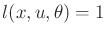, over all,, and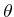.

The expected cost from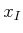is straightforward to compute. With probability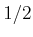, the cost to reach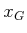is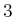. With probability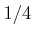, the cost is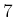. With probability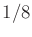, the cost is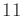. Each time another cycle is taken, the cost increases by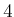, but the probability is cut in half. This leads to the infinite series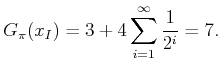(10.47)

The infinite sum is the standard geometric series and converges to; hence (10.47) converges to.

Even though the cost converges to a finite value, this only occurs in the limit. An infinite number of value iterations would theoretically be required to obtain this result. For most applications, an approximate solution suffices, and very high precision can be obtained with a small number of iterations (e.g., after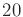iterations, the change is on the order of one-billionth). Thus, in general, it is sensible to terminate the value iterations after the maximum cost-to-go change is less than a threshold based directly on precision.

Note that if nondeterministic uncertainty is used, then the value iterations do not converge because, in the worst case, nature will cause the state to cycle forever. Even though the goal is not guaranteed reachable, the probabilistic uncertainty model allows reasonable solutions.Steven M LaValle 2020-08-14